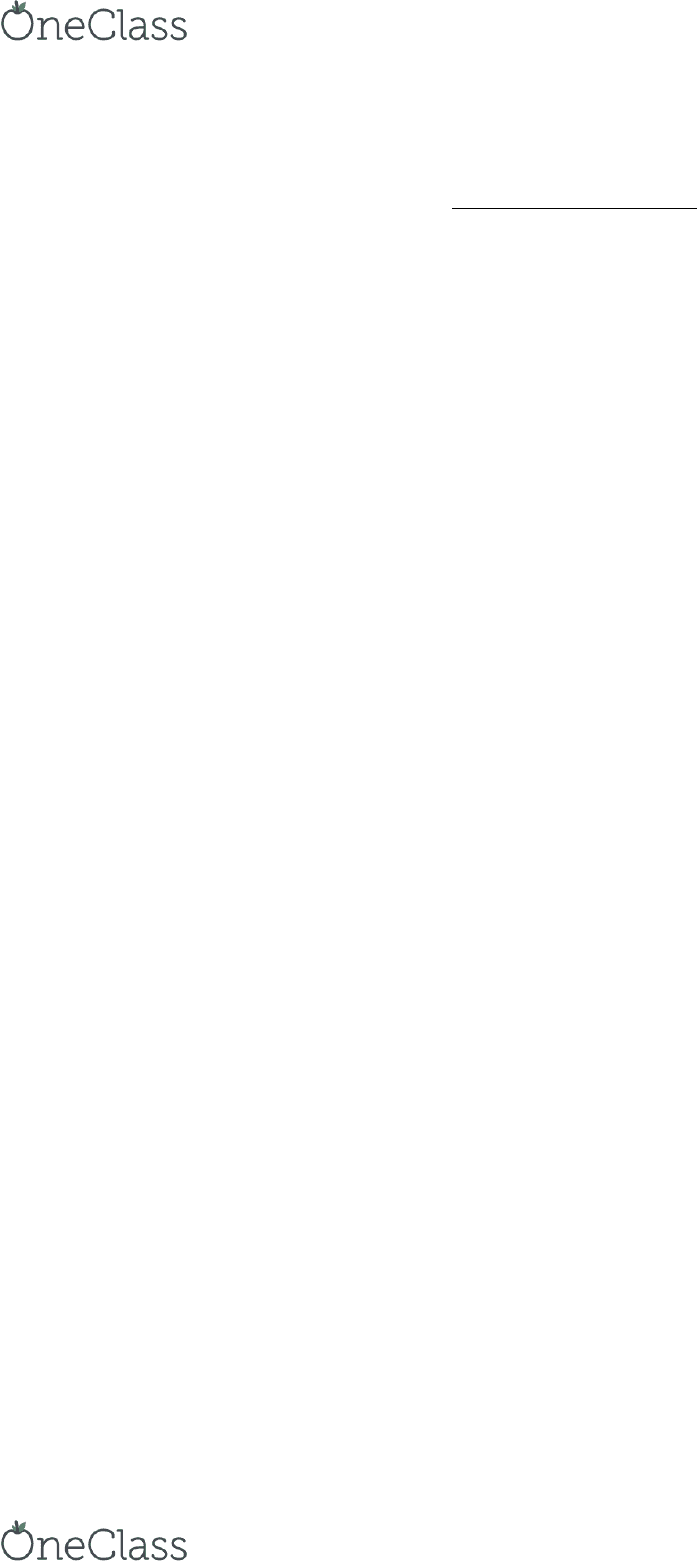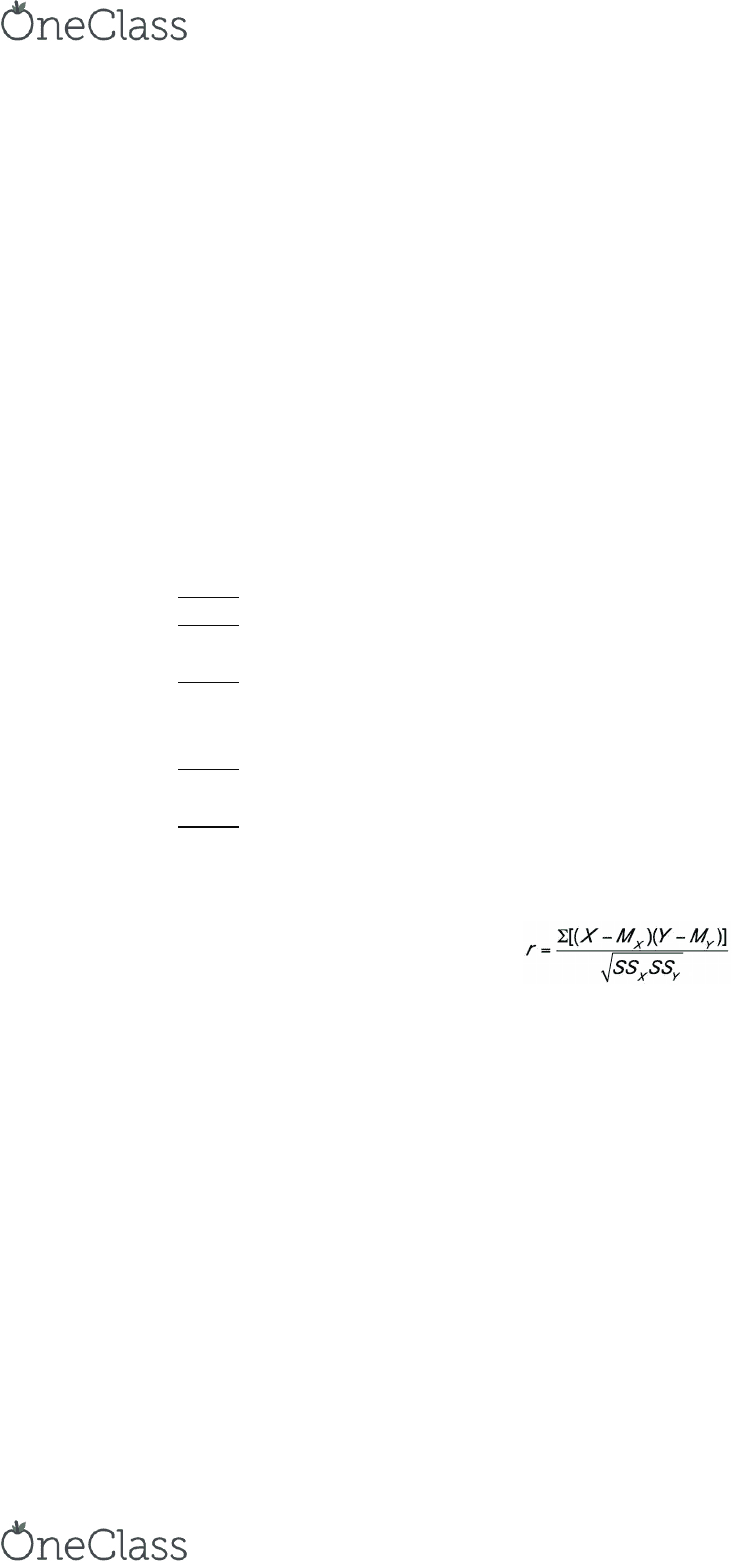Class Notes (1,100,000)
CA (620,000)
Carleton (20,000)
PSYC (3,000)
PSYC 2002 (100)
Lecture 12

# PSYC 2002 Lecture Notes - Lecture 12: Simple Linear Regression, Linear Regression, Standard Score

Department
Psychology
Course Code
PSYC 2002
Professor
Angel Mackenzie
Lecture
12

This preview shows pages 1-3. to view the full 14 pages of the document.Thursday, March 31st, 2016
PSYC 2002 – Regression
Lecture Outline:
- Regression as prediction
- Relationship b/w correlation & regression
- Conceptual basis of regression
- Regression lines on scatterplots
- Multiple regression
- Regression – NH Chapter 14
Regression and correlation defined:
- Correlation: measure of degree of relationship b/w 2 variables
- Regression: how well variation in 1 variable can predict variation in another variable
Linear Regression:
- If we know value for X, can we predict what corresponding value of Y will be?
- Ex. Does knowing how many hrs person studies predict final exam grades? Does lots of
study predict higher grades?
Equation for a graphing a line:
-Ŷ = bX + a (aka Y = mX + b)
-X = variable used to do prediction (predictor variable)
-b = slope of line
oSlope of regression line = rise/run
oRise/run = Y distance / X distance
-a = y-intercept
-Ŷ = variable that’s predicted (dependent variable)
- *graph in lecture slide*
Relationship between regression and correlation:
- Regression & correlation related to each other
- Conceptually: if 2 variables related to each other, then knowing value of 1 variable
should allow you to make prediction about value of other variable
- Mathematically: r & Z-scores
Mathematical relationship between correlation and regression:
-Y = rxyZX
orxy = coefficient for correlation b/w X & Y
Same “r” we calculated for correlations
find more resources at oneclass.com
find more resources at oneclass.com

Only pages 1-3 are available for preview. Some parts have been intentionally blurred.Note: only true for simple linear regression (i.e., Pearson r coefficient isn’t
regression coefficient for multiple regression…)
oY = predicted Z score
- Collectively known as prediction model or standardized regression equation
Prediction using rxy & X-scores:
-Y = rxyZX
- Prediction using this formula is in terms of Z-scores
oFor given Z-score for X, you can predict Z-score for Y
oRemember that we’re standardizing (using Z-scores) in case X & Y values use
different metrics
Regression calculations: Example *in lecture slide*
Regression calculations:
- Step 1: calculate r
- Step 2: select 2 values of X (i.e., predictor variable) & transform them into their
corresponding Z-scores
- Step 3: insert Z-scores for predictor variable (separately) in standardized regression
equation (multiply by r)
oCalculating 2 separate values of Y
- Step 4: take resulting Y & transform into Ŷ
oTransforming Y “back” into raw (predicted) score of Y
- Step 5: add X & Ŷ coordinates (2 pairs) to scatterplot
Regression calculations: Example
- Step 1: calculate r
oAlready done: rxy = -0.962
- Step 2: select 2 X values to transform to Z values; use ZX = (X-MX)/SDX
oOriginal Z-score formula from way back when…
oX = 1 & X = 20
o1 → (1 – 6.3)/4.9
ZX1 = -1.082
o20 → (20 – 6.3)/4.9
ZX2 = 2.796
- Insert ZX-values into standardized regression equation: Y = rxyZX (where rxy = -.0962)
oY = (-0.962)(-1.082)
oFor X =1, Y = 1.040
oY = (-0.962)(2.796)
oFor X =20, Y = -2.689
- These Y values need to be transformed back into predicted raw scores
- Use Ŷ = [(Y)(SDY)] + MY
oŶ1 = [(1.040)(16.907)] + 62.6
find more resources at oneclass.com
find more resources at oneclass.com

Only pages 1-3 are available for preview. Some parts have been intentionally blurred.= 80.189
oŶ2 = [(-2.689)(16.907)] + 62.6
= 17.135
- Thus, X-Y pairs required to plot the line are: (1, 80.189), (20, 17.135)
- Recap: how did we get these values?
- Chose 2 values of X (preferably at “opposite” ends of range of X) & converted these
values to Z-scores
oX1 = 1, X2 = 20
oZX = (X-MX)/SDX
- Plugged those Z-scores into standard regression equation & calculated 2 values of Y
oY = rxyZX
- Converted both values of Y back to raw scores:
oŶ = [(Y)(SDY)] + MY
- This provides us w/ predicted values of Y (80.189 & 17.135) that we can plot w/ their
corresponding Z values (1 & 20)
Regression Line:
- Once prediction model has been generated, regression line can be added to scatter plot
oRegression line based on X & Y pairs (selected values of X & their corresponding
predicted values of Y, Ŷ) – line can simply be drawn “connecting the dots”
oNeed minimum of two “sample” X values (& corresponding predicted Y values) to
determine line
- *graph in lecture slide*
- Regression line = “line of best fit”
oLine that best represents pattern of relationship b/w X & Y
- Regression line may or may not correspond to actual values; predicted range of values
based on what’s known about actual X & Y values
Simple linear regression equation:
- Can now calculate equation for regression line (given our 2 points)
- Simple linear regression equation, Ŷ = bX + a, can be obtained as 2 parts: slope & Y-
intercept
- Slope can be calculated using X-Ŷ pairs used to draw regression line on scatterplot
Regression Line: b (slope)
- Slope = “rise/run” = Y-distance/X-distance
- Slope of regression line can be calculated by taking ratio of difference b/w 2 X-values &
2 Ŷ-values
Regression Line: a (Y-intercept)
- Same process as finding values of Ŷ but we use X = 0
- Insert X-value for zero (0) into standardized regression equation: Y = rxyZX
oUse ZX = (X-MX)/SDX
(0 - 6.3)/4.9
find more resources at oneclass.com
find more resources at oneclass.com
###### You're Reading a Preview

Unlock to view full version